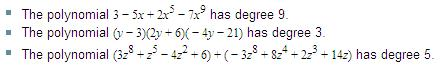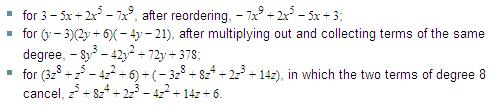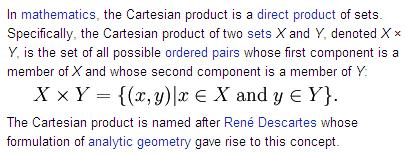Knowing Which Rings Are FieldsB A S I C   A L G E B R AAlgebraic Structures

The goal of this chapter is to ensure you aware of the fact that particular rings are not fields (such as integers, polynomial rings, and matrix rings) and that you thotoughly understand what that means – which obviously requires you to know what a ring is in the first place. So, let’s find out.“”‘’

Many sets are naturally endowed with two binary operations: addition and multiplication.

And of course, a binary operation is an operation (or calculation) that applies to two input (or quantities). Examples of such sets are the integers, the integers modulo n, the real numbers, matrices, and polynomials.

When considering these sets as groups, we simply used addition and ignored multiplication. In many instances, however, one wishes to take into account both addition and multiplication. One abstract concept that does this is the concept of a ring. The notion of a ring originated with Richard Dedekind in the mid-nineteenth-century. though the actual term was coined in 1897 by the German mathematician David Hilbert (1862-1943), and its first formal abstract definition was not given until Abraham Fraenkel presented it in 1914.

DEFINITION
A ring R is a set with two binary operations, addition (denoted by a + b) and multiplication (denoted by ab), such that for all a, b, c in R:

1.   a + b = b + a (commutative under addition)

2.   (a + b) + c = a + (b + c) associative (by definition of a group)

3.   There is an element 0 in R such that a + 0 = a (by definition of a group)

4.   There is an element –a in R such that a + (–a) = 0 (by definition of a group)

5.   a(bc) = (ab)c (associative under multiplication)

6.   a(b + c) = ab + ac and (b + c)a = ba + ca (the left and right distributivelaws hold for all elements of R
EXAMPLES OF RINGS:

• The rational, real and complex numbers form (commutative) rings. (In fact, they are even fields. [Every field is by definition a commutative ring]).
• The polynomial ring R[X] of polynomials over a ring R is also a ring.

• The set of natural numbers N is NOT a ring, since (N, +) is not even a group (the elements are not all invertible with respect to addition). For instance, there is no natural number which can be added to 3 to get 0 as a result.

POLYNOMIAL RING

In abstract algebra, a polynomial ring is the set of polynomials in one or more variables with coefficients in a ring.

Degree of Polynomial

The degree of a polynomial is the maximum of the degrees of all terms in the polynomial. For example, in 2x³ + 4x² + x + 7, the term of highest degree is 2x3; therefore the polynomial is said to have degree 3. Sometimes the same concept is called the order of the polynomial.

If in addition a*b=b*a for all a and b in R, R is called a commutative ring. If R is a commutative ring with the property that all nonzero elements have multiplicative inverses (i.e. for all a there exists a b in R such that a*b = 1 = b*a) then R is a field. The integers Z are a commutative ring but are NOT a field because the only elements in Z that have multiplicative inverses are 1 and -1. The elements in R that have multiplicative inverses are called units. Even though the integers are not a field, they do have the cancellation property:
So a ring is an Abelian group under addition. (In mathematics, an abelian group, also called a commutative group, is a group (G, * ) such that a * b = b * a for all a and b in G. In other words, the order of elements in a product doesn't matter. Such groups are generally easier to understand, although large infinite abelian groups remain a subject of current research.).

So, a ring is in Abelian group under addition, also having an associative multiplication that is left and right distributive over addition.

Note that multiplication need not be commutative!

When multiplication is commutative, we say that the ring is commutative.

Also, a ring need not have an identity under multiplication.  When the ring other than {0} has an identity under multiplication, we say that the ring has a unity (or identity).

A nonzero element of a commutative ring with unity need not hae a multiplicative inverse. When it does, we say that it is a unit of the ring. Thus a is a unit if a¯¹ exists.

The following terminology and notation are convenient.  If a and b belongs to a commutative ring R and a is nonzero, we say that a divides b (or that a is a factor of b) and write a l b, if there exists an element c in R such that b = ac. If a does not divide b, we write ab.

For an abstraction to be worthy of study, it must have many diverse concrete realizations.  The following list of examples shows that the ring concept is pervasive.
l
Integers are an example of an integral domain. In abstract algebra, an integral domain is a commutative ring with 0 ≠ 1 in which the product of any two non-zero elements is always non-zero. Integral domains are generalizations of the integers and provide a natural setting for studying divisibility.In general, to determine the degree of a polynomial expression, the expression has to be brought in "canonical form" by multiplying out until all terms are a product of constants and variables, in which terms with the same product of variables are collected together, and terms in which the constant factor is zero are elided. Usually (but not necessarily) the terms are also ordered from highest to lowest degree. The canonical forms of the three examples above are:

Degree function in abstract algebra

Given a ring R, the polynomial ring R[x] is the set of all polynomials in x that have coefficients chosen from R. In the special case that R is also a field, then the polynomial ring R[x] is a principal ideal domain and, more importantly to our discussion here, a euclidean domain.

It can be shown that the degree of a polynomial over a field satisfies all of the requirements of the norm function in the euclidean domain. That is, given two polynomials f(x) and g(x), the degree of the product f(x)•g(x) must be larger than both the degrees of f and g individually. In fact, something stronger holds:

deg( f(x) • g(x) ) = deg(f(x)) + deg(g(x))
For an example of why the degree function may fail over a ring that is not a field, take the following example. Let R = , the ring of integers modulo 4. This ring is not a field (and is not even an integral domain) because 2•2 = 4 (mod 4) = 0. Therefore, let f(x) = g(x) = 2x + 1. Then, f(x)•g(x) = 4x2 + 4x + 1 = 1. Thus deg(f•g) = 0 which is not greater than the degrees of f and g (which each had degree 1).

Since the norm function is not defined for the zero element of the ring, we consider the degree of the polynomial f(x) = 0 to also be undefined so that it follows the rules of a norm in a euclidean domain.In mathematics, a ring is an algebraic structure in which addition and multiplication are defined and have properties listed below. A ring is a generalization of the integers. Other examples include the polynomials and the integers modulo n. The branch of abstract algebra which studies rings is called ring theory.

Formal definition
A ring is a set R equipped with two binary operations + : R × R → R and · : R × R → R (where × denotes the Cartesian product), called addition and multiplication, such that:

• (R, +) is an abelian group with identity element 0 (identity)
• For every a in R, there exists an element denoted −a, such that a + −a = −a + a = 0 (inverse)
• (R, ·) is a monoid with identity element 1: (a·b)·c = a·(b·c) 1·a = a·1 = a (associative under  multiplication)
• Multiplication distributes over addition: (left and right distributive laws hold for all elements) a·(b + c) = (a·b) + (a·c)  (a + b)·c = (a·c) + (b·c)EXAMPLES OF GROUPS

• the integers
• the rational numbers
• the real numbers
• and the complex numbers under addition
• non-zero rationals
• reals
• complex numbers under multiplication
• non-singular matrices under multiplication
• the group of invertible functions under composition.
• if a is nonzero and a*b = a*c then b = c.

A commutative ring with this property is called an integral domain. The cancellation property is equivalent to the ring not having any zero divisors. I.E. if a*b = 0 then either a=0 or b=0.
EXAMPLES OF GROUPS:

A familiar group is the group of integers under addition.

Integers are not a group under multiplication.
If we consider the integers with the operation of multiplication, denoted by "·", then (Z,·) is not a group. It satisfies most of the axioms, but fails to have inverses:

It is not true that whenever a is an integer, there is an integer b such that ab = ba = 1. For example, a = 2 is an integer, but the only solution to the equation ab = 1 in this case is b = 1/2. We cannot choose b = 1/2 because 1/2 is not an integer. (Inverse element fails) Since not every element of (Z,·) has an inverse, (Z,·) is not a group.

Abelian groups
A group G is said to be an abelian group (or commutative) if the operation is commutative, that is, for all a, b in G, a * b = b * a. A non-abelian group is a group that is not abelian. The term "abelian" is named after the mathematician Niels Abel.